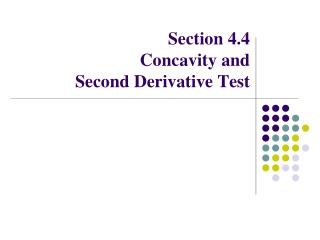DownloadDownload PresentationSection 4.4 Concavity and Second Derivative Test

# Section 4.4 Concavity and Second Derivative Test

Download Presentation## Section 4.4 Concavity and Second Derivative Test

- - - - - - - - - - - - - - - - - - - - - - - - - - - E N D - - - - - - - - - - - - - - - - - - - - - - - - - - -
##### Presentation Transcript

1. Section 4.4 Concavity and Second Derivative Test

2. Definition • A function f (x) is concave up on an interval (a,b) if the graph of f (x) lies above its tangent line at each point on (a,b). • A function f (x) is concave down on an interval (a,b) if the graph of f (x) lies below its tangent line at each point on (a,b). • A point where a graph changes its concavity is called an inflection point. • If the slopes of the tangent lines are increasing, then the function is concave up. • If the slopes of the tangent lines are decreasing, then the function is concave down. Facts

3. Test For Concavity • If f ’’(x) > 0 on an interval (a,b), then f (x) is concave up on (a,b). • If f ’’(x) < 0 on an interval (a,b), then f (x) is concave down on (a,b). • If f ’’(x) changes signs at c, then f(x) has an inflection point at c. Example: Find the intervals where the function is concave up or concave down, and find all inflection points

4. How to find inflection points and interval of concavity: • Find all numbers c such that f ’’(c) = 0 or f ’’(c) is undefined. • Put all values found in step 1 on the number line and use test values to determine the sign of the second derivative for each interval. • Determine the interval of concavity based on the sign of the second derivative.

5. Examples Find the intervals of concavity and all inflection points.

6. Second Derivative test Let c be a critical number of a function f (x) . • If f ’’(c) > 0, then f (x) has a local minimum at c. • If f ’’(c) < 0, then f (x) has a local maximum at c. • If f ’’(c) = 0 or dne, then this test fails  must use the first derivative test! Example: Find all local extrema

7. Examples • a) Find the intervals where the function is increasing/decreasing, and find all local extrema. • b) Find the intervals where the function is concave up or concave down, and find all inflection points